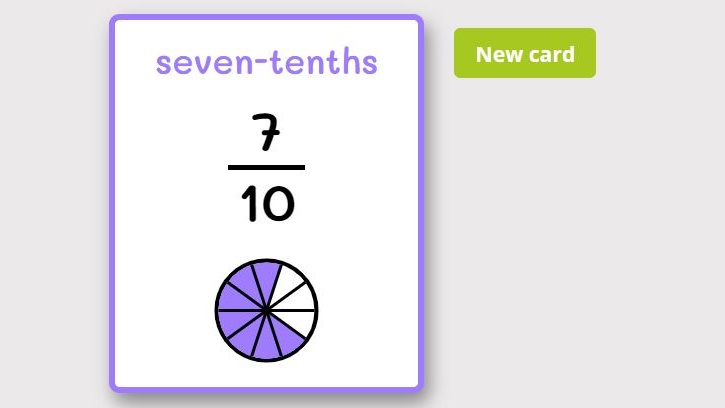﻿ FREE Fraction Flash Cards ONLINE + Printable

Page No. 1030

# Fraction flash cards

Free fraction flash cards online. Equivalent fraction cards free. Fraction Loop Cards. Fraction vocabulary cards PDF. Online fraction flashcards games. Fraction task cards. printable fraction circles. Free flashcards fractions online. Flash cards fractions terminology. Free fraction cards printable free. fraction cards with pictures. Printable fraction flash cards printable. fraction shapes printable. Free printable fraction cards PDF.

## FREE Fraction Flash Cards ONLINEEnjoy our online fraction flash cards. I created these fractions cards to provide fun and easy practice of fractions. Press the button "New card" and a new fraction flash card will appear on the screen.

## Printable fraction flashcards PDF

Click the image bellow and download free printable fraction flashcards in PDF. Our printable fraction cards range from 1/10 to 10/10. Cut and fold these fractions flashcards in such a way that a fraction remains on one side and a circle on the other:

You can find more fractions worksheets here.

## Online fractions cards

Our online fraction flash cards are online math cards that help students learn about fractions. These fraction cards show a fraction with a corresponding textual description and visual representation.

For example, a fraction flash card might display the fraction "1/2", textual description "one-half", and a picture of a circle cut into two equal parts, with one part shaded in.

Fraction flash cards can be used in a variety of ways to help students learn about fractions, including matching games, memory games, and drills: you can ask students to identify the fraction, convert it to a decimal or percentage, try to place fractions on a number line or add and subtract fractions.

Fractions cards are especially useful for students who are struggling with fractions or who need extra practice to master the concept.

Our online fraction flash cards can be used for learning names of fractions in English as well.## Online fraction flashcards

Our online fraction flash cards use visual aids, circles or fraction bars, to introduce students the concept of fractions.

These flashcards of fractions are generated automatically, so every time a new fraction card is created in the range from 1 (1/1) to 10/10.

### Online fraction cards

Our online fraction flash cards are online fraction games that can be played anywhere and anytime.

Try also these online math flash cards:

## How to use fraction flash cards for learning fractions

Fraction flashcards can be a useful tool for introducing and learning fractions.

When using fraction cards during math lessons it is useful to start with the basic fractions such as halves, thirds, and quarters (fourths) before moving on to more complex fractions.

It is also important to use fraction flashcards regularly, because repetition is key to mastering the concept.

### Printable fraction cards vs. online fraction flashcards

There are many fractions apps and online resources (as this one) available that use fraction flashcards to teach the concept of fractions. Consider using these tools in addition to physical printable fraction flashcards.

Both types of fractions flashcards can help you to make the fraction practice more fun.

By using fraction flashcards in a variety of ways, kids can gain a deeper understanding of fractions and develop the skills needed to work with them confidently.

## How to learn fractions using fraction loop cards

• First of all, students learn to identify fractions: Fractions can be identified by their shape, which is a horizontal line between two numbers. The number above the line is the numerator and the number below the line is the denominator.
• Introducing the relationship between parts and whole numbers: Using fraction cards kids can see that numerator represents the number of parts you have and the denominator represents the total number of parts.

Besides fraction flashcards you can practice identifying and working with fractions using our fraction worksheets, fraction games, or real-life examples.

#### What are fractions?

Fractions are a mathematical concept used to represent a part of a whole or a ratio between two quantities.

A fraction consists of two parts: the numerator and the denominator. The numerator represents the number of parts being considered, while the denominator represents the total number of equal parts that make up the whole.

For example, the fraction 3/4 can be interpreted as "three out of four equal parts." In this case, the numerator is 3, which represents the number of parts being considered, and the denominator is 4, which represents the total number of parts that make up the whole.

Fractions can be used to represent many real-world situations, such as dividing a pizza into equal slices or calculating a percentage. Fractions can be added, subtracted, multiplied, and divided just like whole numbers, but they may require some additional steps to simplify or convert them to a common denominator.

Category: